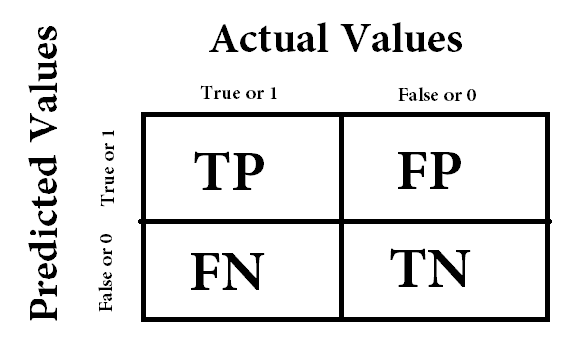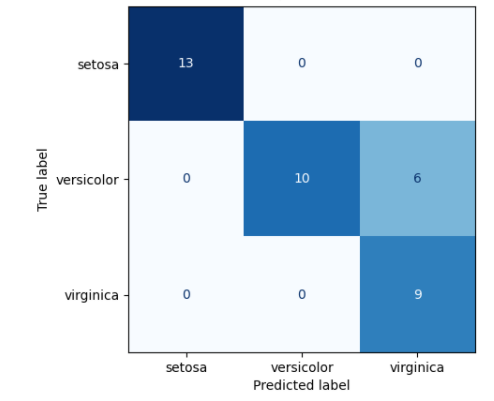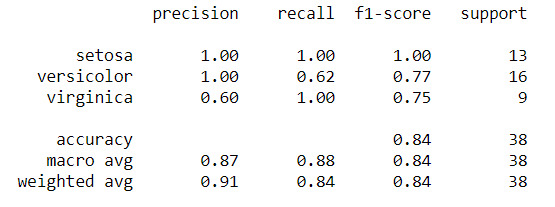HomeBlogArtificial IntelligenceDemystifying Confusion Matrix in Machine Learning [Astonishing]

# Demystifying Confusion Matrix in Machine Learning [Astonishing]

Last updated:
7th Dec, 2020
Views
5 MinsView AllMachine Learning is an umbrella term under which it accumulates Data processing, deriving meaningful insights from the Data, and Data modeling. After being done with Data modeling, the model needs to be measured for its performance evaluation, and other industry-based parameters and one of the most common metrics is the Confusion Matrix.

## Best Machine Learning and AI Courses Online

 Master of Science in Machine Learning & AI from LJMU Executive Post Graduate Programme in Machine Learning & AI from IIITB Advanced Certificate Programme in Machine Learning & NLP from IIITB Advanced Certificate Programme in Machine Learning & Deep Learning from IIITB Executive Post Graduate Program in Data Science & Machine Learning from University of Maryland To Explore all our courses, visit our page below. Machine Learning Courses

## What is the Confusion Matrix and Why it is used?

It is a performance metric used in classification problems where the output classes maybe two or more and this matrix represents all the combinations of predicted values by a model with actual values associated with that input. It’s called ‘confusion matrix’ because going by the definitions it seems easy, but as we move forward to derive more valuable parameters, confusion arises regarding which parameter is best suited at a particular place.

It is used in places where the classification problem is highly imbalanced and one class dominates over other classes. In such scenarios, you may be surprised to see the accuracy of the model peaking at 99% but in reality, the model is highly biased towards the dominant class. There is very little possibility that you will get predictions for minority classes. Therefore, to test such an imbalanced dataset, we consider the confusion matrix.

## In-demand Machine Learning Skills

 Artificial Intelligence Courses Tableau Courses NLP Courses Deep Learning Courses

Get Machine Learning Certification from the World’s top Universities. Earn Masters, Executive PGP, or Advanced Certificate Programs to fast-track your career.

## Structure of the Confusion Matrix

The size of the matrix is directly proportional to the number of output classes. It is a square matrix where we assume the column headers as actual values and the row headers as model predictions. The values which are true and predicted true by the model are True Positives (TP), correct negative value predictions are True Negatives (TN), values which were negative but predicted as true are False Positives (FP) and positive values predicted as negative are False Negatives (FN). Have a look at this image:## What can we learn from this?

A valid question arises that what we can do with this matrix. There are some important terminologies based on this:

1. Precision: It is the portion of values that are identified by the model as correct and are relevant to the problem statement solution. We can also quote this as values, which are a portion of the total positive results given by the model and are positive. Therefore, we can give its formula as TP/ (TP + FP).
2. Recall: It is the portion of values that are correctly identified as positive by the model. It is also termed as True Positive Rate or Sensitivity. Its formula comes out to be TP/ (TP+FN).
3. F-1 Score: It is the harmonic mean of Precision and Recall. It means that if we were to compare two models, then this metric will suppress the extreme values and consider both False Positives and False Negatives at the same time. It can be quoted as 2*Precision*Recall/ (Precision+Recall).
4. Accuracy: It is the portion of values that are identified correctly irrespective of whether they are positives or negatives. It means that all True positives and True negatives are included in this. The formula for this is (TP+TN)/ (TP+TN+FP+FN).

Out of all the terms, precision and recall are most widely used. Their tradeoff is a useful measure of the success of a prediction. The desired model is supposed to have high precision and high recall, but this is only in perfectly separable data. In practical use cases, the data is highly unorganized and imbalanced.

## How to create code for Confusion Matrix in Python?

The sklearn library provides a variety of functionalities to perform all the machine learning tasks with utmost accuracy and almost everything has been implemented here. Consider the famous Iris dataset with all import statements already done, the code for confusion matrix would be:

X = iris.data

y = iris.target

class_names = iris.target_names

X_train, X_test, y_train, y_test = train_test_split(X, y, random_state=0)

classifier = svm.SVC(kernel=‘linear’, C=0.01).fit(X_train, y_train)

plot_confusion_matrix(classifier, X_test, y_test,display_labels=class_names,cmap=plt.cm.Blues)Note: The matrix returned by this has reversed sides, here on the left we have actual values and on the top, we have predicted values. If you want to avoid confusion, execute this function to get a detailed summary (classification report)  instead of calculating it manually:

print(classification_report(y_true=y_test, y_pred=y_pred, target_names=class_names))## Which one to use and where?

This is the most common question that arises while modeling the Data and the solution lies in the problem’s statement domain. Consider these two cases:

1. Suppose you are predicting whether the person will get a cardiac arrest. In this scenario, you can’t afford any misclassification and all the predictions made should be accurate. With that said, the cost of False Negatives is high, so the person was prone to attack but was predicted as safe. These cases should be avoided. In these situations, we need a model with high recall.
2. Suppose a search engine provided random results that are all predicted as positive by the model, then there is very little possibility that the user will rely on it. Therefore, in this scenario, we need a model with high precision so that user experience improves, and the website grows in the right direction.

## Conclusion

The confusion matrix is a great method to evaluate a classification model. It gives the actual insight into how accurately the model has classified the classes based upon the inputs provided and how this class can be misclassified.

If you’re interested to learn more about machine learning, check out IIIT-B & upGrad’s PG Diploma in Machine Learning & AI which is designed for working professionals and offers 450+ hours of rigorous training, 30+ case studies & assignments, IIIT-B Alumni status, 5+ practical hands-on capstone projects & job assistance with top firms.

## Popular AI and ML Blogs & Free Courses

 IoT: History, Present & Future Machine Learning Tutorial: Learn ML What is Algorithm? Simple & Easy Robotics Engineer Salary in India : All Roles A Day in the Life of a Machine Learning Engineer: What do they do? What is IoT (Internet of Things) Permutation vs Combination: Difference between Permutation and Combination Top 7 Trends in Artificial Intelligence & Machine Learning Machine Learning with R: Everything You Need to Know AI & ML Free Courses Introduction to NLP Fundamentals of Deep Learning of Neural Networks Linear Regression: Step by Step Guide Artificial Intelligence in the Real World Introduction to Tableau Case Study using Python, SQL and TableauBlog Author
Director of Engineering @ upGrad. Motivated to leverage technology to solve problems. Seasoned leader for startups and fast moving orgs. Working on solving problems of scale and long term technology strategy.
Get Free Consultation

Select CourseSelectBy clicking 'Submit' you Agree to

## Suggested Blogs

91233
AWS Projects & Topics Looking for AWS project ideas? Then you’ve come to the right place because, in this article, we’ve shared multiple AWS proj